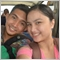# How to close all orders when price reaches take profit?29

Hi all! I've been trying to close all orders with my custom function CloseAllOrders  which works fine but cant seem to be triggered with the condition if(Bid==OrderTakeProfit()) as seen below in my code.

What seems to be the problem? hoping for your help on this guys. Thanks.

``` for(b=OpenOrdersThisPair(Symbol())-1;b>=0;b--)
{
if(OrderSymbol()==Symbol())
{

OrderModify(OrderTicket(),OrderOpenPrice(),OrderOpenPrice()-((PipsBasis-1)*pips), OrderOpenPrice()+(PipsBasis*pips),0,CLR_NONE);
if(Bid==OrderTakeProfit())
{
CloseAllOrders();
}```20207

1. Don't double post. Your other post has been deleted.
2. Doubles are rarely equal.
The == operand. - MQL4 and MetaTrader 4 - MQL4 programming forum
3. The purpose of the take profit is to close orders automatically. You will never find a buy order with Bid==OrderTakeProfit.29

Thank you for sharing the informative forum links whroeder1. After reading them all, what I have understood is that it is not necessary to use the Normalizedouble and i tried to use digits on my trigger. I tried test it but it still did not work.
```   for(int i=0; i<OrdersTotal();i++)
{
{
if(OrderSymbol()==Symbol())
{
{
if(Bid>=OrderTakeProfit()+Point&&Bid<=OrderTakeProfit()+(1*pips)-Point)
{
CloseAllOrders();
}

}
if(OrderType()==OP_SELL)
{
{
CloseAllOrders();
}
}
}
}
}```

What I'm trying to do is to have a trigger at Buy when price reaches between the TP and TP+1pip and at Sell between TP and TP-1 but it was not triggered.

Also would like to ask what are the values on the journal tab beside the TP with ()?

2018.03.07 19:18:24.392 2016.12.28 13:15:03  Tester: take profit #3 at 1.04289 (1.04276 / 1.04289)

Thank you for your patience and guidance.20207

What you're trying to do will never work. № 1.329

Would it be ok to ask what can be a workaround in this case? what functions can be used?20207

When in doubt, think. There are no "functions to be used." Remember the TP, wait for the order to be close and then open.29

I'll keep that in mind sir.

Thank you for your time and ideas whroeder1. It is much appreciated.29

Hi everybody! ive changed my logic as based on whroeder1's guidance on comparing doubles.Thanks you point it out. But Im still trying to create my Hedging mechanism EA so here goes my second shot. Everyones advice and guidance would be much appreciated. So here goes.

My EA tries to do the below.

1. When there is an Opened Order, a hedge is opened at the opposite direction with double the lot size. So only 2 orders are created-This works fine.

2. If TP is hit, All remaining orders are  closed to make a new start. This also works fine but in the process of refining it.

3. If SL is hit. The pending order is the basis of creating a new pending order on the other end. i.e if buystop is left, a sellstop is created. both having the same lotsize.

4. If either of them becomes an open order, the pending order's lotsize is doubled.

What does not work as expected here is step 3. Below is my code

```void LowRiskHedge()
{
//initialize variables to zero
int b=0;
int b2=0;
int s=0;
int SelectedTicket=0;
double SellPrice=0;
double SellLotSize=0;
double SellstopPrice=0;
double SellstopLots=0;
int PendingOrder=0;
int OpenOrder=0;
bool ModifyStatus=True;
int Ticket=0;
bool Delete=True;

if(OpenOrdersThisPair(Symbol())==1)
{
for(b=OpenOrdersThisPair(Symbol())-1;b>=0;b--)
{
if(OrderSymbol()==Symbol())
SelectedTicket=OrderTicket();
{
Print("Enter Process 1" );
ModifyStatus=OrderModify(OrderTicket(),OrderOpenPrice(),OrderOpenPrice()-((PipsBasis-1)*pips), OrderOpenPrice()+(PipsBasis*pips),0,CLR_NONE);
ErrHndlingOrderModify(ModifyStatus);
ErrHndlngOrderSend(Ticket);
}
else if(OrderType()==OP_SELL&&OrderStopLoss()==0&&OrderTakeProfit()==0)
{
Print("Enter Process 2" );
ModifyStatus=OrderModify(OrderTicket(),OrderOpenPrice(),OrderOpenPrice()+((PipsBasis-1)*pips), OrderOpenPrice()-(PipsBasis*pips),0,CLR_NONE);
ErrHndlingOrderModify(ModifyStatus);
SellPrice=OrderOpenPrice();
SellLotSize=OrderLots();
ErrHndlngOrderSend(Ticket);
}
else if((OrderType()==OP_BUYSTOP||OrderType()==OP_SELLSTOP)&&(ClosedOrderProfit2()>0))//We Close the Hedge since we earned some profit and we can enter another trading opportunity
{
Print("Enter Process 3" );
Delete=OrderDelete(SelectedTicket);
ErrHndlingOrderDelete(Delete);
}
else if((OrderType()==OP_BUYSTOP||OrderType()==OP_BUY)&&(ClosedOrderProfit2()<0))//We hedge and double the lotsize since we exited at a loss
{
Print("Enter Process 4" );
ErrHndlngOrderSend(Ticket);
}
else if((OrderType()==OP_SELLSTOP||OrderType()==OP_SELL)&&(ClosedOrderProfit2()<0))//We hedge and double the lotsize since we exited at a loss
{
Print("Enter Process 5" );
SellPrice=OrderOpenPrice();
SellLotSize=OrderLots();
ErrHndlngOrderSend(Ticket);
}else Print("Error: Process Not Implemented");

}
}

int ClosedOrderProfit2()// This checks if exit is via TP or SL
{
int ClosedProfit=0;

for(int Count=OrdersHistoryTotal()-1;Count>=0;Count--)
{

if(OrderSelect(Count,SELECT_BY_POS,MODE_HISTORY))
{
if(OrderSymbol()==Symbol())
{
if(OrderProfit()>0)ClosedProfit++;
else if(OrderProfit()<0)ClosedProfit--;
break;
}
}
}
return(ClosedProfit);

}```

My problem here is that "Process 5" is not executed after a stoploss which I am expecting it to do.This is shown in order #5 in my testing.

Results from the testing is shown below.

Ive specifically gave the conditions to create a hedge if there is a Sellstop or a sell in the order pool in process 5 but it did not do it despite the fact that there was a Sellstop left after the SL of order #5.

It instead waited for the SellStop To become a sell before it entered the process. What went wrong and what should I do?

Hoping for some guidance on this. Thank you!